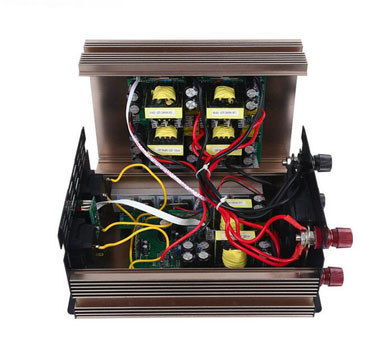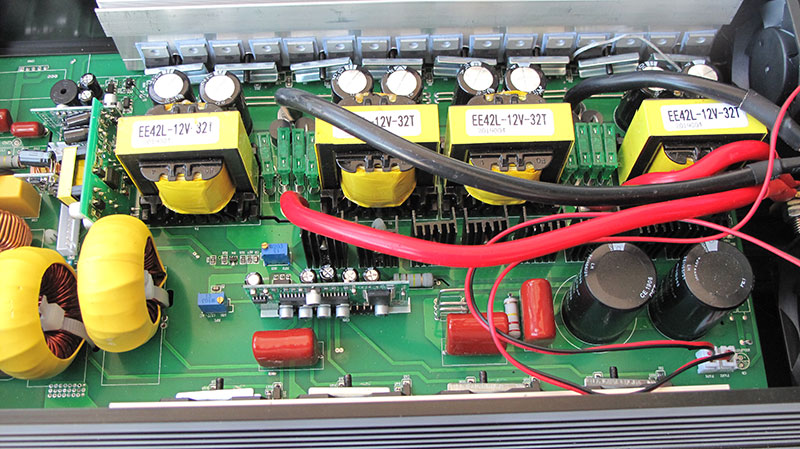Close

# How to Improve Efficiency of Power Inverters?

The efficiency of the power inverter is directly related to the generated energy of the system, so it is an important indicator that customers care much about. It is of great importance to increase the conversion efficiency of the power inverter.

The only way to improve the efficiency of power inverters is to reduce the losses. The main losses of inverters come from IGBT, MOSFET and other power switch tubes, as well as magnetic devices such as transformers and inductors, which is related to the current, voltage and the process adopted by the selected materials.

 Component Type Factors Measures IGBT Switching loss (dynamic) Switching frequency, DC voltage Multi-level, control strategy Conduction loss (static) Current, component internal resistance Soft switching, new component Inductance Iron loss (no-load loss) Capacity, magneto resistance Improve switching frequency and magnetic conductive material Copper loss (load loss) Current, internal resistance Good conductive materials

IGBT loss

It can be divided into conduction loss and switching loss. Among them, conduction loss is related to internal resistance and current passing through components, while switching loss is related to switching frequency of components and DC voltage endured by components.Inductance loss

It can be divided into copper loss and iron loss. Among them, copper loss refers to the loss caused by resistance of inductance coil. When current is heated by passing through the resistance of inductance coil, part of electric energy is transformed into heat energy and lost, and because the coil is usually wrapped by insulated copper wire, it is also called copper loss, which can be calculated by measuring the short-circuit impedance of transformers. Iron loss includes two aspects: hysteresis loss and eddy current loss, which can be calculated by measuring the no-load current of transformers.

Techniques to improve the efficiency of inverters

At present, there are three technical routes to improve the efficiency of power inverters.

• First, space vector pulse width modulation and other control methods are used to reduce loss.
• Second, components of silicon carbide materials are used to reduce the internal resistance of power devices.
• Third, three-level, five-level, multi-level electrical topology and soft switching technology are adopted to reduce the voltage at both ends of power devices and the switching frequencies of power devices.

1. Space vector pulse width modulation (SVPWM)

SVPWM is a fully digital control method with the advantages of high utilization rate of DC voltage and easy control, which has been widely used in power inverters. With high utilization of DC voltage, lower DC bus voltage can be used under the same output voltage, which reduces the voltage stress of power switching devices, reduces the switching loss on devices and improves the conversion efficiency of power inverters to a certain extent. In space vector synthesis, there are many combinations of vector sequences. Through different combinations and sorting, the effect of reducing switching times of power devices can be achieved, which can further reduce the switching losses of power devices of power inverters.